Next: Tests Up: Detection of High Curvature Previous: Beus and Tiu BT87

# The new algorithm

The proposed two-pass algorithm defines a corner in a simple and intuitively appealing way, as a location where a triangle of specified size and opening angle can be inscribed in a curve. A curve is represented by a sequence of points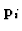in the image plane. The ordered points are densely sampled along the curve, but, contrary to the other four algorithms, no regular spacing between them is assumed. A chain-coded curve can also be handled if converted to a sequence of grid points. In the first pass the algorithm scans the sequence and selects candidate corner points. The second pass is post-processing to remove superfluous candidates.

First pass. In each curve point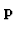the detector tries to inscribe in the curve a variable triangle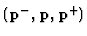constrained by a set of simple rules: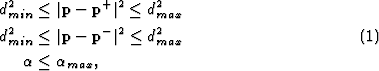where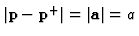is the distance betweenand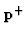,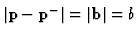the distance betweenand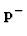, and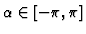the opening angle of the triangle. (See figure 1a.) The latter is computed as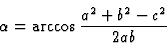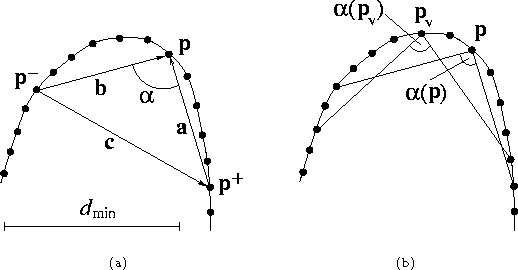Variations of the triangle that satisfy the conditions (7) are called admissible. Search for the admissible variations starts fromoutwards and stops if any of the conditions (7) is violated. (That is, a limited number of neighboring points is only considered.) Among the admissible variations, the least opening angle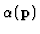is selected.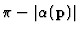is assigned toas the sharpness of the candidate. If no admissible triangle can be inscribed,is rejected and no sharpness is assigned.

Considering the vector product of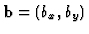and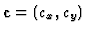, it is easy to see that the corner is convex if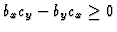, otherwise it is concave.

Second pass. The sharpness based non-maxima suppression procedure is illustrated in figure 1b. A corner detector can respond to the same corner in a few consecutive points. Similarly to edge detection, a post-processing step is needed to select the strongest response by discarding the non-maxima points.

A candidate pointis discarded if it has a sharper valid neighbor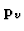: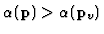. In the current implementation, a candidate pointis a valid neighbor ofif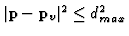. As alternative definitions, one can use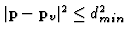or the points adjacent to.

Parameters.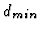,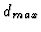and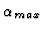are the parameters of the algorithm.sets the scale (resolution), with small values responding to fine corners. The upper limitis necessary to avoid false sharp triangles formed by distant points in highly varying curves.is the angle limit that determines the minimum sharpness accepted as high curvature. In practice, we often set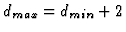and tune the remaining two parameters,and. The default values are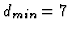and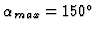.Next: Tests Up: Detection of High Curvature Previous: Beus and Tiu BT87
Dmitry Chetverikov
1999-04-26